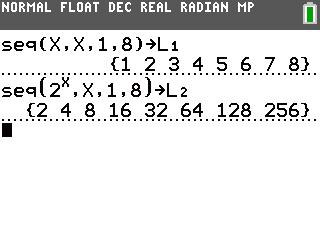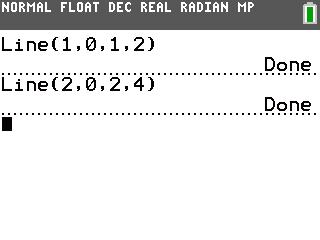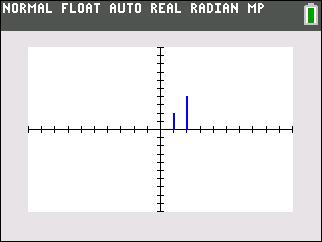•• Device
• TI-83 Plus Family
• TI-84 Plus
•TI-84 Plus CE
• Report an Issue

SA and NT: Double Tree
by Texas InstrumentsOverview

Students visually explore geometric sequences by modeling the growth of a tree that doubles in height every year.

Key Steps

•In this activity, students model tree growth using the sequence 2n. They use the Line command to draw line segments, representing the height of a tree. The commands shown in the screenshot, draws lines from (1, 0) to (1, 2) and (2, 0) to (2, 4).

•Students complete a table describing the x-value of the line, height of the tree, and the power of two for the years 2001 to 2008.Students complete a table describing the x-value of the line, height of the tree, and the power of two for the years 2001 to 2008.

•Then students use the seq command to create a scatter plot the sequence 2n. The first sequence, stored in L1, contains the integers 1 to 8, representing the years. The second sequence, stored in L2, contains the values of the sequence 2n, representing the heights of the tree.